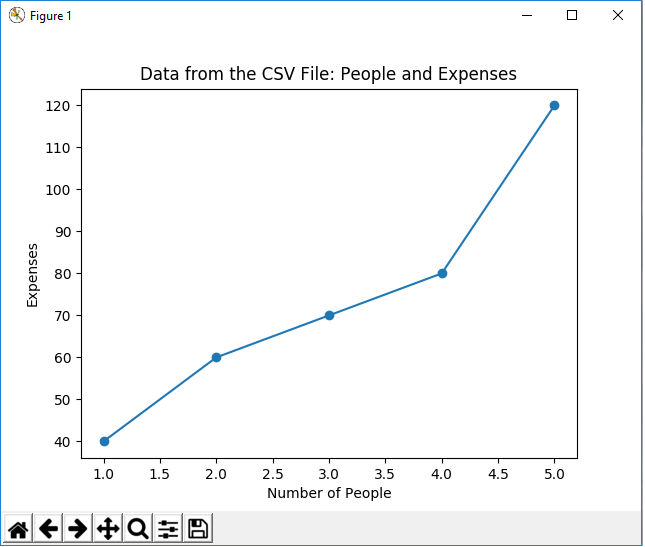﻿ How to Plot a Graph with Matplotlib from Data from a CSV File using the CSV Module in Python ﻿# How to Plot a Graph with Matplotlib from Data from a CSV File using the CSV Module in PythonIn this article, we show how to plot a graph with matplotlib from data from a CSV file using the CSV module in Python.

So basically you won't always be plotting graphs straight up from a Python IDLE by typing in that data.

Many times, the data that you want to graph is found in some type of file, such as a CSV file (comma-separated values file).

Using the CSV module in Python, we can import a CSV file, read it, and extract the data from it, such as the x-axis data and the y-axis data.

We can then use matplotlib in order to plot the graph of the extracted data.

So what we have to do is create a CSV file. This can either be done with a high-level software such as Microsoft Excel or it can be done with a simple editor such as notepad. In this example, I actually create my CSV file with notepad. I save it with a .txt file extension.

I create a very basic CSV file, consisting of an x-axis that goes from 1 to 5.

These 5 data points have y-axis values.

This CSV file is shown at the following link: Example CSV File

Remember to place this CSV file in the folder that Python is running in. To see which folder this is, import the os module and type in, os.getcwd()

This will get the current directory that Python is operating in.

Place the CSV file in this directory, or change the directory to another one using the os.chdir() function.

So below, we read and extract data from this CSV file and then plot the data using matplotlib.

So the first thing we have to do is import matplotlib. We do this with the line, import matplotlib.pyplot as plt

We then have to import the csv module, since we use this module in order to read a CSV file. This is done using the line, import csv

We then have the variable, x, which we set equal to an empty list.

We then have the variable, y, which we set equal to an empty list, as well.

We then are able to open the CSV file with the line, with open('csvfile1.txt', 'r') as csvfile.

This is like a file handle. It doesn't read the file, but it allows us to open the file to be able to read it. 'r' means read. This file handle is reference by csvfile.

We then have a variable, plots, which we set equal to, csv.read(csvfile, delimiter=',')

We then have a for loop that goes through each rows of the plot data.

The delimiter is a comma, so data gets separated by the comma found in the CSV file.

We append to the variable, x, which is a list using the append() function. Into this function, we pass in, int(row)

This takes the data before the comma on each line and appends it to the x variable.

We then append to the variable, y, which is a list using the append() function. Into this function, we pass in, int(row)

This takes the data after the comma on each line and appends it to the y variable.

We now have our x and y variables populated.

This ends the file reading and the extraction of data.

We now go on to focus completely on the plotting of the graph.

We plot a line graph with the plt.plot() function.

We specify x and y and we add a marker so that each data point contains a marker.

We give the graph a title, x label, and y label.

We then show the plot.

Once we run this code, we get the following output shown below.And this is how to plot a graph with matplotlib from a CSV file using the CSV module in Python.

Related Resources

How to Randomly Select From or Shuffle a List in Python